Linear connection

(diff) ← Older revision | Latest revision (diff) | Newer revision → (diff)

A linear connection on a differentiable manifoldis a differential-geometric structure onassociated with an affine connection on. For every affine connection a parallel displacement of vectors is defined, which makes it possible to define for every curveina linear mapping of tangent spaces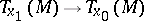. In this sense an affine connection determines a linear connection on, to which all concepts and constructions can be transferred which only depend on the displacement of vectors and, more generally, of tensors. A linear connection onis a connection in the principal bundleof frames in the tangent spaces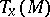,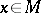, and is defined in one of the following three equivalent ways:

1) by a connection object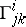, satisfying the following transformation law on intersections of domains of local charts: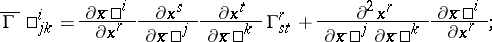2) by a matrix of-forms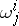on the principal frame bundle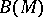, such that the-forms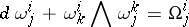in each local coordinate system can be expressed in the form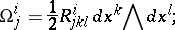3) by the bilinear operator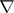of covariant differentiation, which associates with two vector fields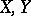ona third vector fieldand has the properties: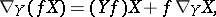whereis a smooth function on.

Every linear connection onuniquely determines an affine connection oncanonically associated with it. It is determined by the involute of any curve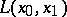in. To obtain this involute one must first definelinearly independent parallel vector fieldsalong, then expand the tangent vector field toin terms of them,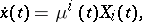and finally find in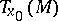the solution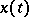of the differential equationwith initial value. At an arbitrary pointofan affine mapping of tangent affine spaces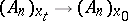is now defined by a mapping of frames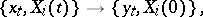where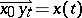.

A linear connection is often identified with the affine connection canonically associated with it, by using the one-to-one correspondence between them.

A linear connection on a vector bundle is a differential-geometric structure on a differentiable vector bundle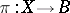which associates with every piecewise-smooth curveinbeginning atand ending at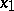a linear isomorphism of the fibres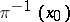and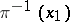as vector spaces, called parallel displacement along. A linear connection is determined by a horizontal distribution on the principal bundleof frames in the fibres of the given vector bundle. Analytically, a linear connection is specified by a matrix of-forms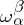on, where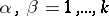, wheredenotes the dimension of the fibres, such that the-formsare semi-basic, that is, in every local coordinate systemonthey can be expressed in the form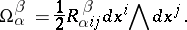The horizontal distribution is determined, moreover, by the differential systemon. The-forms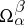are called curvature forms. According to the holonomy theorem they determine the holonomy group of the linear connection.

A linear connection in a fibre bundleis a connection under which the tangent vectors of horizontal curves beginning at a given pointofform a vector subspace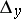of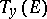; the linear connection is determined by the horizontal distribution: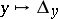.

How to Cite This Entry:
Linear connection. Encyclopedia of Mathematics. URL: http://encyclopediaofmath.org/index.php?title=Linear_connection&oldid=17268
This article was adapted from an original article by Ãœ. Lumiste (originator), which appeared in Encyclopedia of Mathematics - ISBN 1402006098. See original article